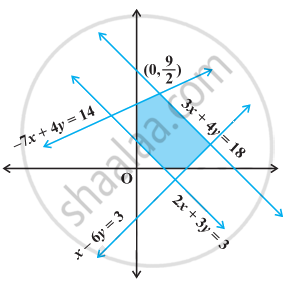# Find the linear inequalities for which the shaded region in the given figure is the solution set. - Mathematics

Sum

Find the linear inequalities for which the shaded region in the given figure is the solution set.

#### Solution1. Consider 2x + 3y = 3. We observe that the shaded region and the origin lie on opposite side of this line and (0, 0) satisfies 2x + 3y ≤ 3. Therefore, we must have 2x + 3y ≥ 3 as linear inequality corresponding to the line 2x + 3y = 3.
2. Consider 3x + 4y = 18. We observe that the shaded region and the origin lie on the same side of this line and (0, 0) satisfies 3x + 4y ≤ 18. Therefore, 3x + 4y ≤ 18 is the linear inequality corresponding to the line 3x + 4y = 18.
3. Consider –7x + 4y = 14. It is clear from the figure that the shaded region and the origin lie on the same side of this line and (0, 0) satisfies the inequality –7x + 4y ≤ 14. Therefore, –7x + 4y ≤ 14 is the inequality corresponding to the line –7x + 4y = 14.
4. Consider x – 6y = 3. It may be noted that the shaded portion and origin lie on the same side of this line and (0, 0) satisfies x – 6y ≤ 3. Therefore, x – 6y ≤ 3 is the inequality corresponding to the line x – 6y = 3.
5. Also the shaded region lies in the first quadrant only. Therefore, x ≥ 0, y ≥ 0. Hence, in view of (i), (ii), (iii), (iv) and (v) above, the linear inequalities corresponding to the given solution set are: 2x + 3y ≥ 3, 3x + 4y ≤ 18 –7x + 4y ≤14, x – 6y ≤ 3, x ≥ 0, y ≥ 0.
Concept: Solution of System of Linear Inequalities in Two Variables
Is there an error in this question or solution?

#### APPEARS IN

NCERT Mathematics Exemplar Class 11
Chapter 6 Linear Inequalities
Solved Examples | Q 9 | Page 104

Share